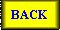# Spring rate and length calculation

-------- Original Message --------
Subject: Spring Rate / Spring Length Calculation
Date: Tue, 26 Feb 2002 05:23:28 -0800
From: Mike Craig
To: sv650-l@LISTSERV.HAWKGT.COM

The formula for spring deflection is:

d = (64)(P)(r*3)(N)/(G)(D*4)

Where d = deflection
D = diameter of the spring material
P = downward force
r = radius of the spring coil
G = modulus
N = the number of active coils
(The active coils equal the total number of coils
minus 1.75 coils.)
r*3 = r raised to the third power
D*4 = D raised to the fourth power

This is an approximation but a very good one.

Knowing that a stock SV spring has a spring rate P/d
of 0.706 kg/mm (from Race Tech); a spring length of
315 mm and 26 coils (12.1 mm per coil) we can reduce
the equation above to something useful.

Let K be a constant equal to (64)(r*3)/(G)(d*4);
substituting into the formula we get

d = (K)(P)/N or P/d = 1/[(K)(N)] or N = 1/[(K)(P/d)]

Remember spring rate is P/d, force per unit distance
deflection. Let's call it SPR. P/d = SPR

So now N = 1/[(K)(SPR)] or K = 1/[(N)(SPR)]

We know spring rate for the stock spring is 0.0706
kg/mm and N is 26-1.75 = 24.25. Therefore

K = 1/[(24.25)(0.706)] = 0.584

>From the above N = 1/[(SPR)(0.0584)]

Total spring length is (N+1.75)(12.1). Let's call it
TL

Therefore for a spring rate of 0.80 the spring length
must be:

N = 1/[(0.80)(0.0584)] = 21.4 active coils

TL = (21.4 +1.75)(12.1) = 280 mm total length

For a spring Rate of 0.85

N = 1/(0.85)(0.0584) = 20.15
TL = (20.15 + 1.75)(12.1) = 265 mm

I have not measured the free space between coils but
keep in mind you will need to maintain enough free
space to equal the fork travel. Don't shorten the
spring too much. My guess is the stock spring is
limited to around 0.95 spring rate. Of course that's
enough for a heavy rider.

Visit Jim Stewart's web site for an excellent method
of cutting the spring (It's not easy) and an equally
good discussion of suspension tuning:
http://www.strappe.com/suspension.html#rate

Please note the constant calculated for the SV(S)650
spring (0.0584) is good only for the stock spring.
Other springs will have a different constant. The
method is valid for other springs but you will have to
know the initial spring rate, length and number of
coils. Then calculate a new constant.

Cheersto Suspension Tuning